Copyright © Michael Richmond. This work is licensed under a Creative Commons License.

# Electric Charge and Coulomb's Law

• Electric charge is a fundamental property of matter. Electrons carry a charge of negative one "electron unit", and protons a charge of positive one "electron unit".
• Electric charge is conserved in a closed system.
• The SI unit for electric charge is the Coulomb:
```            about 6.25 x 10^18  electrons    add up to 1 Coulomb
```
• The Coulomb is a very LARGE amount of charge -- ordinary situations contain a tiny fraction of a Coulomb.
• If one Coulomb per second moves past a fixed point in a wire, that wire is carrying a current of one Ampere.
• Conductors allow charge to move freely through them. Metals are good conductors.
• Insulators keep charged particles nearly fixed in place. Glass, rubber and plastic are good insulators.
• Coulomb's Law describes the force between two charged point-like particles:
```                            q1 * q2
F   =  k * ----------
r^2
```
where
```               k    =  Coulomb's constant = 8.99 x 10^9    (N*m^2/C^2)
q1   =  charge on first particle            (Coulombs)
q2   =  charge on second particle           (Coulombs)
r   =  distance between particles          (meters)
```
If the result is positive, the force is repulsive. If the result is negative, the force is attractive.
• The electric forces from several particles add together as vectors.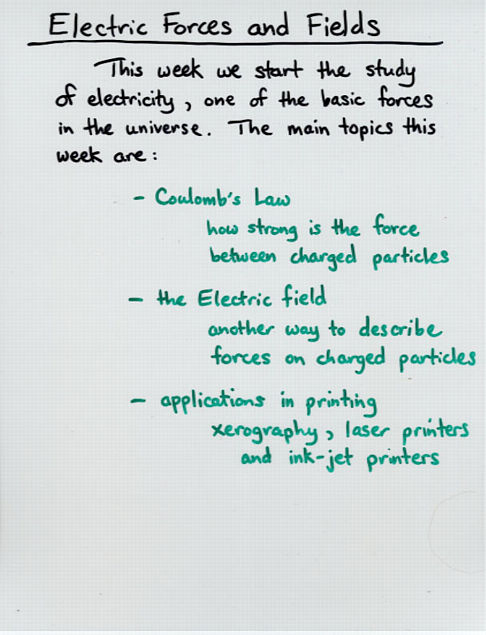Viewgraph 1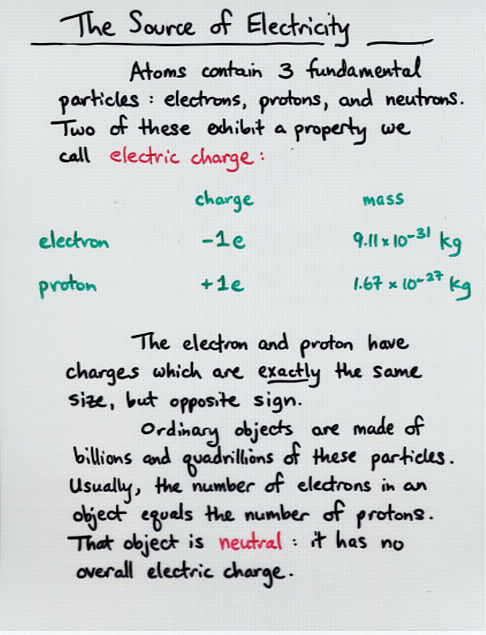Viewgraph 2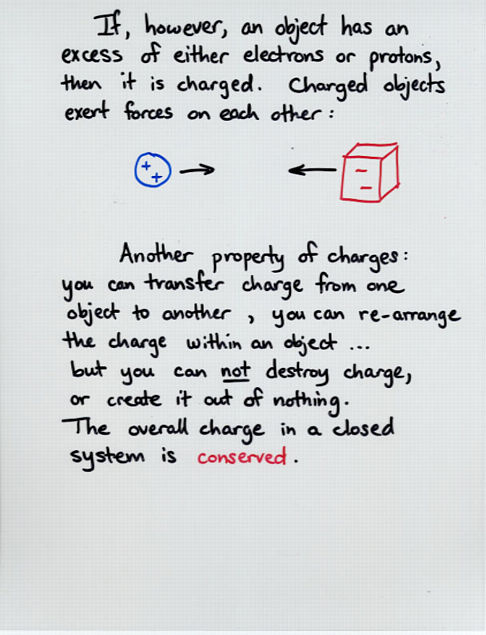Viewgraph 3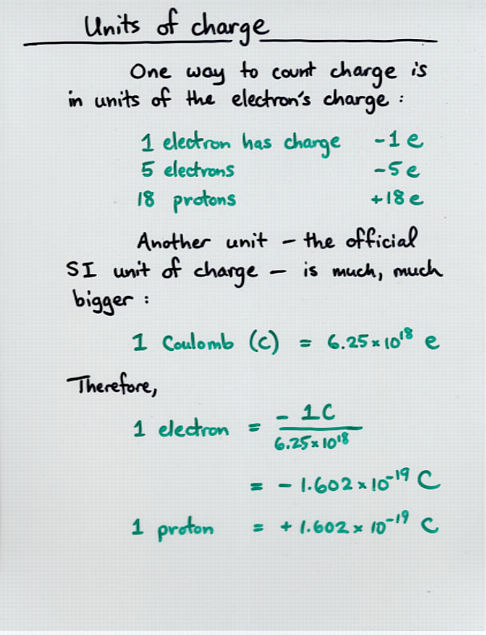Viewgraph 4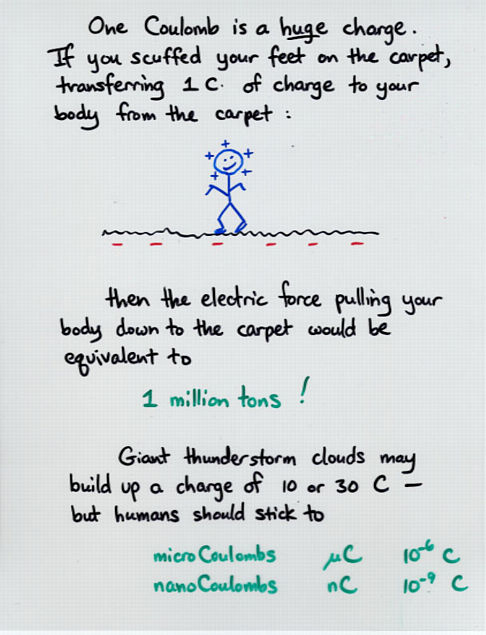Viewgraph 5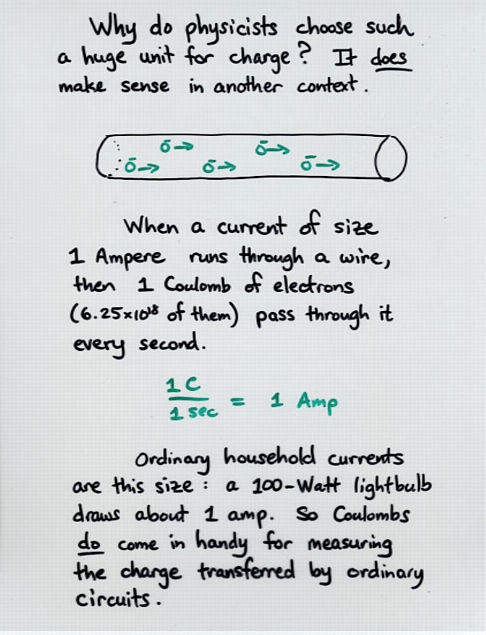Viewgraph 6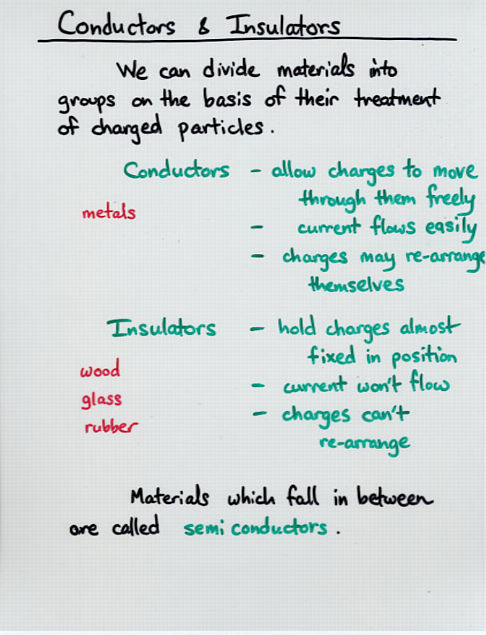Viewgraph 7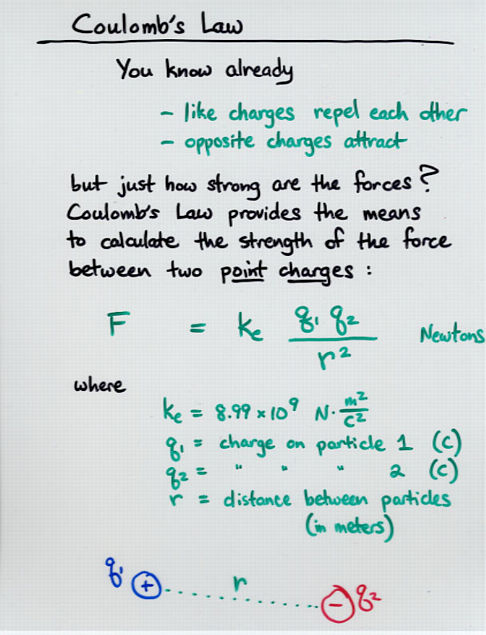Viewgraph 8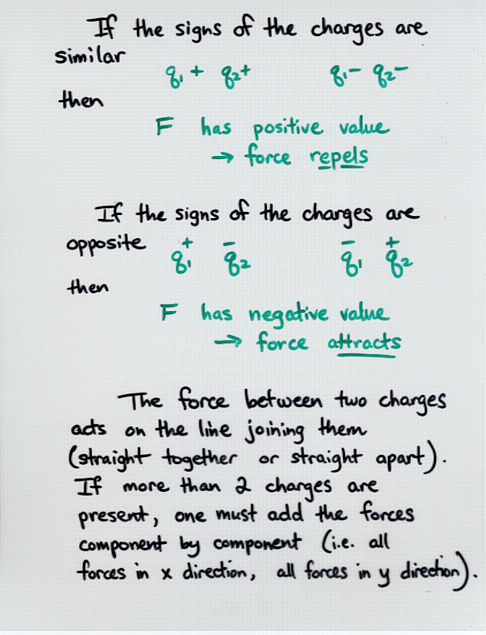Viewgraph 9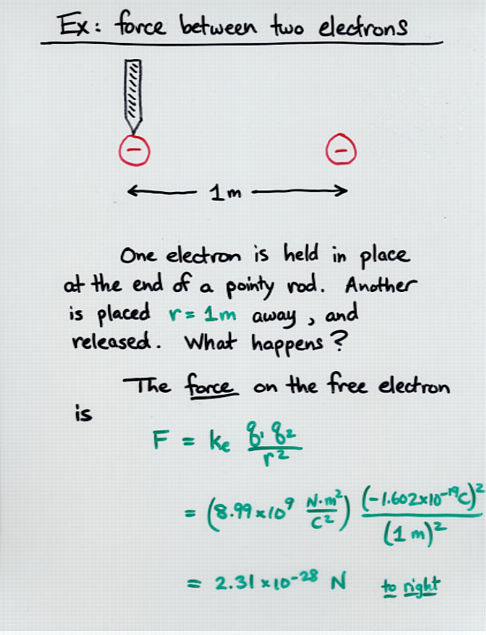Viewgraph 10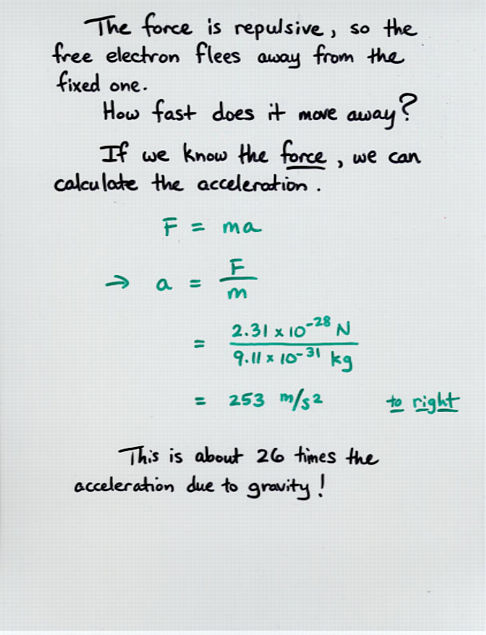Viewgraph 11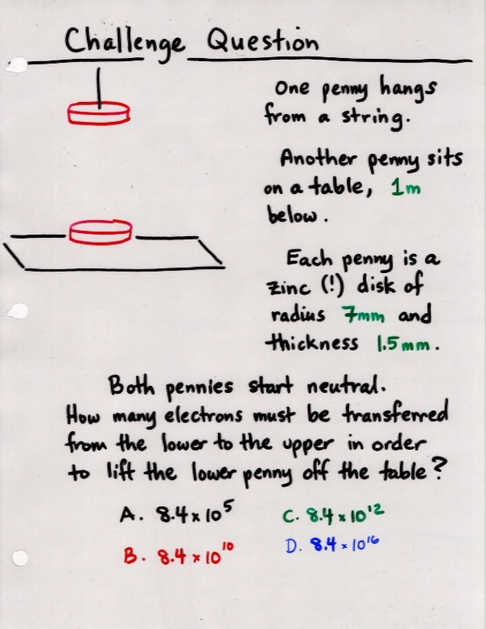Viewgraph 11x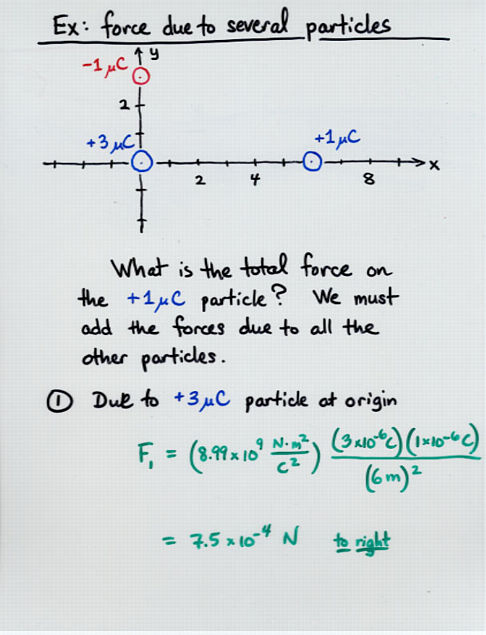Viewgraph 12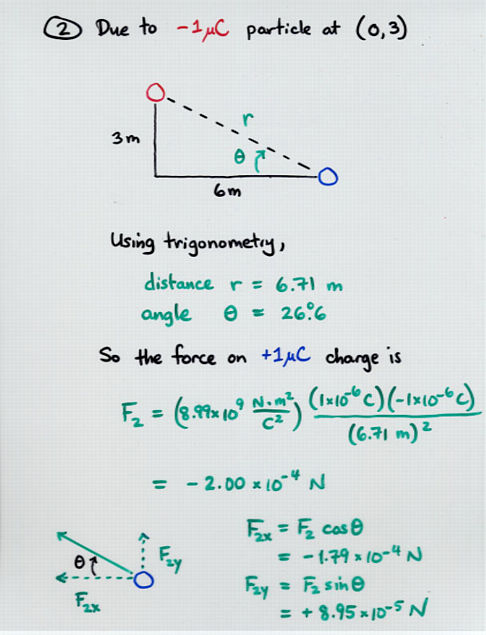Viewgraph 13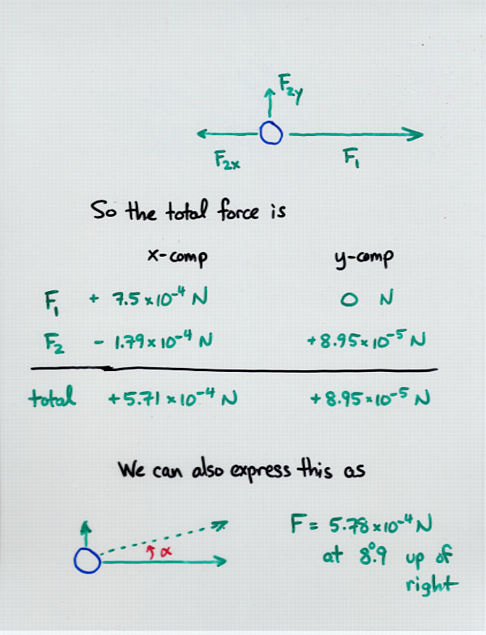Viewgraph 14Copyright © Michael Richmond. This work is licensed under a Creative Commons License.